# Consumption and Investment Functions Notes 12th Economics

12th Economics Lesson 4 Notes in English

4. Consumption and Investment Functions

 The theory of multiplier and the theory of accelerator are the two sides of the theory of fluctuations just as the theory of demand and the theory of supply are the two sides of the theory of value. The full theory must be that which shows both sides in operation. – J.R.Hicks.

Introduction

• In the second chapter we have seen the concept of national income, its measurement, importance and difficulties.
• The present chapter deals with consumption function and the investment function which play a vital role in influencing national income.
• The primary macroeconomic objective is acceleration of growth of national income.
• We have already seen that national income comprises of consumption goods (C) and investment (I) goods.
• There is close correlation between investment and national income.
• The multiplier refers to the change in national income resulting from change in investment.
• The value of multiplier itself depends on consumption function or marginal propensity to consume.
• The consumption function is the relationship between consumption expenditure and the national income.
• The unspent portion of national income is called saving which becomes investment and thereby capital.
• The relationship between consumption expenditure and the capital expenditure is explained by the principle of accelerator.
• All these variables are closely interconnected.
• In this chapter one can learn the consumption function, psychological law of consumption, investment function, multiplier, accelerator and super multiplier.

Consumption Function

Meaning of Consumption Function

• The consumption function or propensity to consume refers to income consumption relationship.
• It is a “functional relationship between two aggregates viz., total consumption and gross national income.”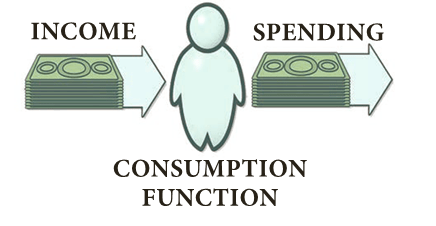• Symbolically, the relationship is represented as C= f (Y). Where,
• C = Consumption Y = Income f = Function
• Thus the consumption function indicates a functional relationship between C and Y, where C is the dependent variable and Y is the independent variable, i.e.,
• C is determined by Y.
• This relationship is based on the ceteris paribus (other things being same) assumption, as only income consumption relationship is considered and all possible influences on consumption are held constant.
• In fact, consumption function is a schedule of the various amounts of consumption expenditure corresponding to different levels of income.
• A hypothetical consumption schedule is given.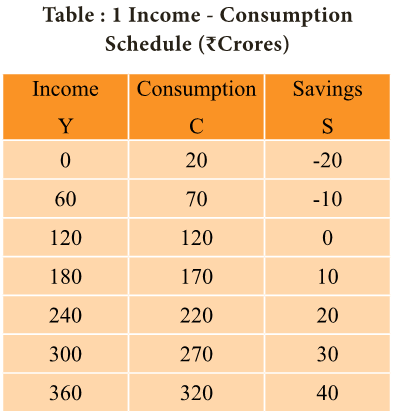• If we take C = 100 + 0.8y, then MPC = 0.8
• Here, if Y = 0, C = 100; if Y = 100, C = 180; if Y = 200, C = 260; if Y = 300, C = 340• In mathematical terms
 C= a + b Y or C = 20 + 0.8Y
• Where a>0 and b<1
• C= Consumption a= constant or intercept = 20 Y= income• The given table shows that consumption is an increasing function of income because consumption expenditure increases with increase in income.
• Here it is shown that when income is zero, people spend out of their past savings on consumption because they must eat in order to live (Autonomous Consumption).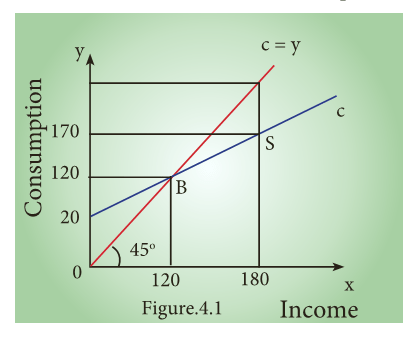• Here, when y = 120, C = 120 (Point B is the diagram)
• When y = 180, C = 170, S = 10 (Point S is the diagram)
• If Y increases to 360, C = 320, S = 40
• In the diagram, income is measured horizontally and consumption is measured vertically.
• In 45 line at all levels, income and consumption are equal.
• It is a linear consumption function based on the assumption that consumption changes by the same amount as does income.
• Thus the consumption function measures not only the amount spent on consumption but also the amount saved.
• This is because the propensity to save is merely the propensity not to consume.
• The 45° line may therefore be regarded as a zero-saving line, and the shape and position of the C curve indicate the division of income between consumption and saving.

Technical Attributes of the Consumption Function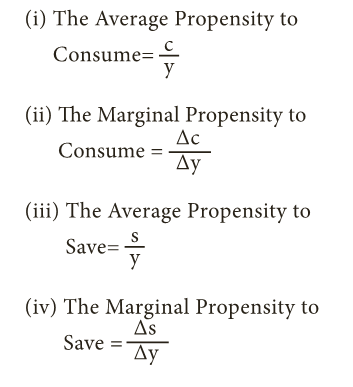(1) The Average Propensity to Consume:

• The average propensity to consume is the ratio of consumption expenditure to any particular level of income.”
• Algebraically it may be expressed as under: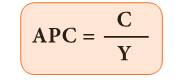• Where, C= Consumption Y = Income

(2) The Marginal Propensity to Consume:

• The marginal propensity to consume may be defined as the ratio of the change in the consumption to the change in income.
• Algebraically it may be expressed as under:(3) The Average Propensity to Save (APS):

• The average propensity to save is the ratio of saving to income.
• APS is the quotient obtained by dividing the total saving by the total income.
• In other words, it is the ratio of total savings to total income.
• It can be expressed algebraically in the form of equation as under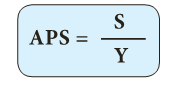• Where, S= Saving ,Y=Income

(4) The Marginal Propensity to Save (MPS) :

• Marginal Propensity to save is the ratio of change in saving to a change in income.
• MPS is obtained by dividing change in savings by change in income.
• It can be expressed algebraically as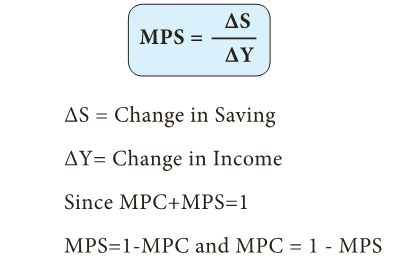• Generally the average ie APC is expressed in percentage and the MPC in fraction.

Calculation of APC, MPC, APS and MPS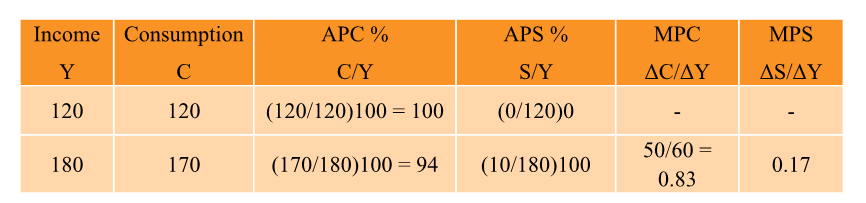Keynes’s Psychological Law of Consumption:

• Keynes propounded the fundamental Psychological Law of Consumption which forms the basis of the consumption function.
• He stated that “The fundamental psychological law upon which we are entitled to depend with great confidence both prior from our knowledge of human nature and from the detailed facts of experience, is that men are disposed as a rule and on the average to increase their consumption as their income increases but not by as much as the increase in their income.”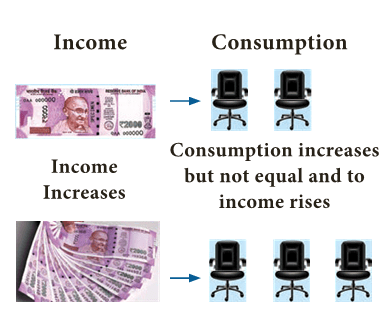• The law implies that there is a tendency on the part of the people to spend on consumption less than the full increment of income.

Assumptions:

Keynes’s Law is based on the following assumptions:

1. Ceteris paribus (constant extraneous variables):

• The other variables such as income distribution, tastes, habits, social customs, price movements, population growth, etc. do not change and consumption depends on income alone.

2. Existence of Normal Conditions:

• The law holds good under normal conditions.
• If, however, the economy is faced with abnormal and extraordinary circumstances like war, revolution or hyperinflation, the law will not operate.
• People may spend the whole of increased income on consumption.

3. Existence of a Laissez-faire Capitalist Economy:

• The law operates in a rich capitalist economy where there is no government intervention.
• People should be free to spend increased income.
• In the case of regulation of private enterprise and consumption expenditures by the State, the law breaks down.

Propositions of the Law:

• This law has three propositions:
1. When income increases, consumption expenditure also increases but by a smaller amount. The reason is that as income increases, our wants are satisfied side by side, so that the need to spend more on consumer goods diminishes. So, the consumption expenditure increases with increase in income but less than proportionately.
2. The increased income will be divided in some proportion between consumption expenditure and saving. This follows from the first proposition because when the whole of increased income is not spent on consumption, the remaining is saved. In this way, consumption and saving move together.
3. Increase in income always leads to an increase in both consumption and saving. This means that increased income is unlikely to lead to fall in either consumption or saving. Thus with increased income both consumption and saving increase.

The Three Propositions of the Law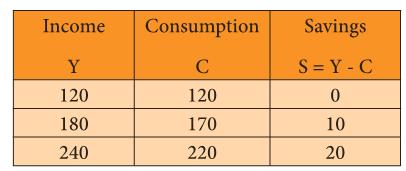Proposition (1):

• Income increases by Rs 60crores and the increase in consumption is by Rs 50crores.

Proposition (2):

• The increased income of Rs 60crores in each case is divided in some proportion between consumption and saving respectively. (i.e., Rs 50crores and Rs 10crores).

Proposition (3):

• As income increases consumption as well as saving increase. Neither consumption nor saving has fallen.
• Diagrammatically, the three propositions are explained in Figure 4.2.
• Here, income is measured horizontally and consumption and saving are measured on the vertical axis.
• C is the consumption function curve and 45° line represents income consumption equality.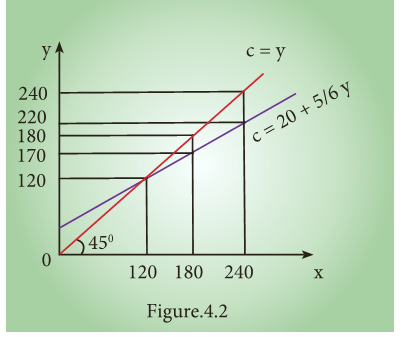Proposition (1):

• When income increases from 120 to 180 consumption also increases from 120 to 170 but the increase in consumption is less than the increase in income, 10 is saved.

Proposition (2):

• When income increases to 180 and 240, it is divided in some proportion between consumption by 170 and 220 and saving by 10 and 20 respectively.

Proposition (3):

• Increases in income to 180 and 240 lead to increased consumption 170 and 220 and increased saving 20 and 10 than before. It is clear from the widening area below the C curve and the saving gap between 45° line and C curve.

Determinants of Consumption function: Subjective and Objective Factors

• J.M Keynes has divided factors influencing the consumption function into two namely: Subjective factors and Objective factors

A) Subjective Factors

• Subjective factors are the internal factors related to psychological feelings.
• Major subjective factors influencing consumption function are given below.
• Keynes lists eight motives which lead individuals to refrain from spending, they are:
1. The motive of precaution: To build up a reserve against unforeseen contingencies. Eg. Accidents, sickness
2. The motive of foresight: The desire to provide for anticipated future needs. Eg. Old age
3. The motive of calculation: The desire to enjoy interest and appreciation.
4. The motive of improvement: The desire to enjoy for improving standard of living.
5. The motive of financial independence.
6. The motive of enterprise (desire to do forward trading).
7. The motive of pride.(desire to bequeath a fortune)
8. The motive of avarice.(purely miserly instinct)
• Keynes sums up the motives as Precaution, Foresight, Calculation, Improvement, Independence, Enterprise, Pride and Avarice.
• The Government, institutions and business corporations and firms may also consume mainly because of the following four motives:
1. The motive of enterprise: The desire to obtain resources to carry out further capital investment without incurring debt.
2. The motive of liquidity: The desire to secure liquid resources to meet emergencies, and difficulties.
3. The motive of improvement: The desire to secure a rising income and to demonstrate successful management.
4. The motive of financial prudence: The desire to ensure adequate financial provision against depreciation and obsolescence and to discharge debt.
• According to Keynes, the subjective factors do not change in the short run and hence consumption function remains stable in the short period.

B) Objective Factors

• Objective factors are the external factors which are real and measurable.
• These factors can be easily changed in the long run.
• Major objective factors influencing consumption function are:

1) Income Distribution

• If there is large disparity between rich and poor, the consumption is low because the rich people have low propensity to consume and high propensity to save.
• The community with more equal distribution of income tends to have high propensity to consume.
• This view has been corroborated by V.K.R.V. Rao.

2) Price level

• Price level plays an important role in determining the consumption function. When the price falls, real income goes up; people will consume more and propensity to save of the society increases.

3) Wage level

• Wage level plays an important role in determining the consumption function and there is positive relationship between wage and consumption.
• Consumption expenditure increases with the rise in wages. Similar is the effect with regard to windfall gains.

4) Interest rate

• Rate of interest plays an important role in determining the consumption function.
• Higher rate of interest will encourage people to save more money and reduces consumption.

5) Fiscal Policy

• When government reduces the tax the disposable income rises and the propensity to consume of community increases.
• The progressive tax system increases the propensity to consume of the people by altering the income distribution in favour of poor.

6) Consumer credit

• The availability of consumer credit at easy installments will encourage households to buy consumer durables like automobiles, fridge, computer.
• This pushes up consumption.

7) Demographic factors

• Ceteris paribus, the larger the size of the family, the grater is the consumption.
• Besides size of family, stage in family life cycle, place of residence and occupation affect the consumption function.
• Families with children of college education stage spend more than those of primary education and urban families spend more than rural families.

8) Duesenberry hypothesis

• Duesenberry has made two observations regarding the factors affecting consumption.1. The consumption expenditure depends not only on his current income but also past income and standard of living. As the individuals are accustomed to a particular standard of living, they continue to spend the same amount on consumption even though the current income is reduced.
2. Consumption is influenced by demonstration effect. The consumption standards of low income groups are influenced by the consumption standards of high income groups.
• In other words, the poor people want to imitate the consumption pattern of rich.
• This results in spending beyond their income level.

9) Windfall Gains or losses

• Unexpected changes in the stock market leading to gains or losses tend to shift the consumption function upward or downward.

Investment Function

• The investment function refers to investment -interest rate relationship.
• There is a functional and inverse relationship between rate of interest and investment.
• The investment function slopes downward.
• I = f (r) I= Investment (Dependent variable) r = Rate of interest (Independent variable)

Meaning of investment

• The term investment means purchase of stocks and shares, debentures, government bonds and equities.
• According to Keynes, it is only financial investment and not real investment.
• This type of investment does result in an addition to the stock of real capital of the nation.
• In the views of Keynes, Investment includes expenditure on capital investment.

Types of investment

• Autonomous Investment and Induced Investment

Autonomous Investment

• Investment that is not dependent on the national income
• Mainly done with the welfare motive and not for making profits
• Examples : Construction of road, bridges, School, Charitable houses
• Not affected by rise in raw materials or wages of workers
• Essential to development of nation and out of depression

i) Autonomous investment:

• Autonomous investment is the expenditure on capital formation, which is independent of the change in income, rate of interest or rate of profit.
• This investment is independent of economic activity.
• Autonomous investment is income-inelastic, the volume of autonomous investment is the same at all levels.
• The autonomous investment curve is horizontal, parallel to X axis.• In the times of economic depression, the governments try to boost the autonomous investment.
• Thus, autonomous investment is one of the key concepts in welfare economics.
• Generally, Government makes autonomous investment because of the welfare consideration.

ii) Induced investment:

• Induced investment is the expenditure on fixed assets and stocks which are required when level of income and demand in an economy goes up.
• Induced investment is profit motivated. It is related to the changes of national income.
• The relationship between the national income and induced investment is positive; decreases in national income leads to decrease in induced investment and vice versa.
• Induced investment is income elastic. It is positively sloped as shown here.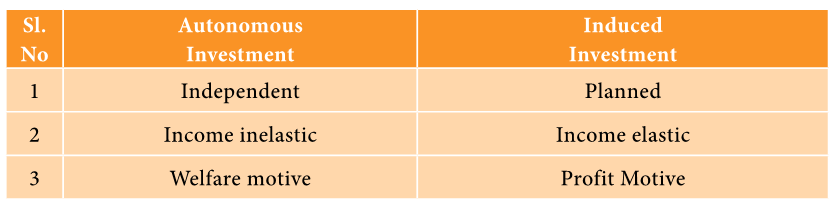Determinants of Investment Function

• The classical economists believed that investment depended exclusively on rate of interest.
• In reality investment decision depends on a number of factors. They are as follows:
1. Rate of interest
2. Level of uncertainty
3. Political environment
4. Rate of growth of population
5. Stock of capital goods
6. Necessity of new products
7. Level of income of investors
8. Inventions and innovations
9. Consumer demand
10. Policy of the state
11. Availability of capital
12. Liquid assets of the investors
• However, Keynes contended that business expectations and profits are more important in deciding investment.
• He also pointed out that investment depends on MEC (Marginal Efficiency of Capital) and rate of interest.
1. Private investment is an increase in the capital stock such as buying a factory or machine.
2. The marginal efficiency of capital (MEC) states the rate of return on an investment project. Specifically, it refers to the annual percentage yield (output) earned by the last additional unit of capital.
3. If the marginal efficiency of capital is 5% and interest rates is 4%, then it is worth borrowing at 4% to get an expected increase in output of 5%.

Relationship between rate of interest and Investment:

• An explanation of how the rate of interest influences the level of investment in the economy.
• Typically, higher interest rates reduce investment, because higher rates increase the cost of borrowing and require investment to have a higher rate of return to be profitable.

Interest rates and investment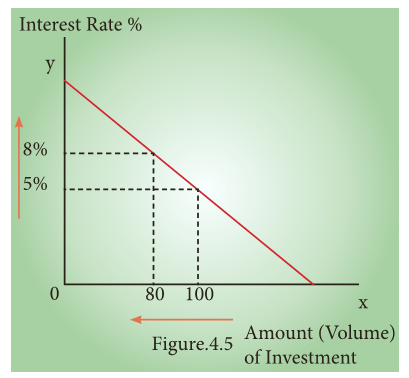• As the real cost of borrowing rises, fewer investment projects are profitable.
• If interest rates rise from 5% to 8 %, then we get a fall in the amount of investment from Rs 100cr to Rs 80cr.
• If interest rates are increased then it will tend to discourage investment because investment has a higher opportunity cost.
1. With higher rates, it is more expensive to borrow money from a bank.
2. Saving money in a bank gives a higher rate of return.
• Therefore, using savings to finance investment has an opportunity cost of lower interest payments.
• If interest rates rise, firms will need to gain a better rate of return to justify the cost of borrowing using savings.

Marginal Efficiency of Capital.

• MEC was first introduced by J.M Keynes in 1936 as an important determinant of autonomous investment.
• The MEC is the expected profitability of an additional capital asset.
• It may be defined as the highest rate of return over cost expected from the additional unit of capital asset.
• Meaning of Marginal Efficiency of Capital (MEC) is the rate of discount which makes the discounted present value of expected income stream equal to the cost of capital.

MEC depends on two factors:

1. The prospective yield from a capital asset.
2. The supply price of a capital asset.

Factors Affecting MEC: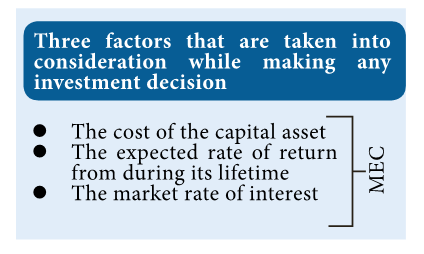• The marginal efficiency of capital is influenced by short – run as well as long- run factors.
• These factors are discussed in brief:
• Three factors that are taken into consideration while making any investment decision

a) Short – Run Factors

(i) Demand for the product:

• If the market for a particular good is expected to grow and its costs are likely to fall, the rate of return from investment will be high.
• If entrepreneurs expect a fall in demand for goods and a rise in cost, the investment will decline.

(ii) Liquid assets:

• If the entrepreneurs are holding large volume of working capital, they can take advantage of the investment opportunities that come in their way.
• The MEC will be high.

(iii) Sudden changes in income:

• The MEC is also influenced by sudden changes in income of the entrepreneurs.
• If the business community gets windfall profits, or tax concession the MEC will be high and hence investment in the country will go up.
• On the other hand, MEC falls with the decrease in income.

(iv) Current rate of investment:

• Another factor which influences MEC is the current rate of investment in a particular industry.
• If in a particular industry, much investment has already taken place and the rate of investment currently going on in that industry is also very large, then the marginal efficiency of capital will be low.

(v) Waves of optimism and pessimism:

• The marginal efficiency of capital is also affected by waves of optimism and pessimism in the business cycle.
• If businessmen are optimistic about future, the MEC will be likely to be high.
• During periods of pessimism the MEC is under estimated and so will be low.

b) Long – Run Factors

• The long run factors which influence the marginal efficiency of capital are as follows:

(i) Rate of growth of population:

• Marginal efficiency of capital is also influenced by the rate of growth of population.
• If population is growing at a rapid speed, it is usually believed that the demand of various types of goods will increase.
• So a rapid rise in the growth of population will increase the marginal efficiency of capital and a slowing down in its rate of growth will discourage investment and thus reduce marginal efficiency of capital.

(ii) Technological progress:

• If investment and technological development take place in the industry, the prospects of increase in the net yield brightens up.
• For example, the development of automobiles in the 20th century has greatly stimulated the rubber industry, the steel and oil industry etc.
• So we can say that inventions and technological improvements encourage investment in various projects and increase marginal efficiency of capital.

(iii) Monetary and Fiscal policies:

• Cheap money policy and liberal tax policy pave the way for greater profit margin and so MEC is likely to be high.

(iv) Political environment:

• Political stability, smooth administration, maintenance of law and order help to improve MEC.

(v) Resource availability:

• Cheap and abundant supply of natural resources, efficient labour and stock of capital enhance the MEC.

Marginal Efficiency of Investment

• MEI is the expected rate of return on investment as additional units of investment are made under specified conditions and over a period of time.
• When cost of borrowing is high, businesses are less motivated to borrow money and make investment on different projects because high cost of borrowing reduces profit margin of the business firms;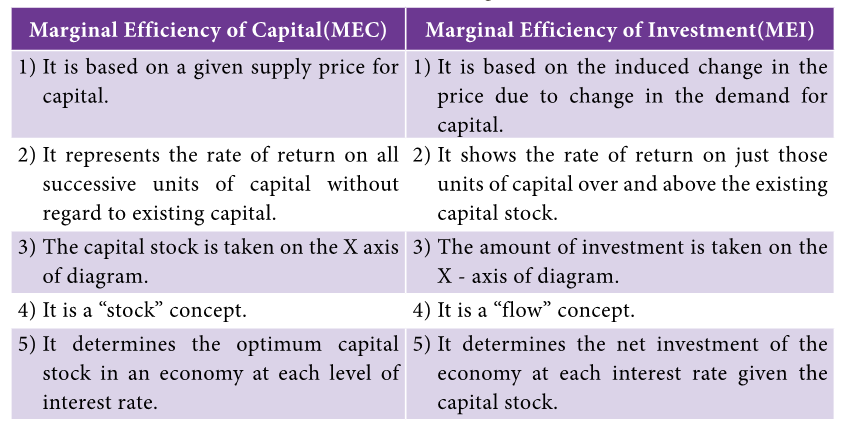Multiplier

• The concept of multiplier was first developed by R.F. Khan in terms of employment.
• J.M Keynes redefined it as investment multiplier.
• The multiplier is defined as the ratio of the change in national income to change in investment.Assumptions of Multiplier:

• Keynes’s theory of the multiplier works under certain assumptions which limit the operation of the multiplier. They are as follows:
1. There is change in autonomous investment.
2. There is no induced investment
3. The marginal propensity to consume is constant.
4. Consumption is a function of current income.
5. There are no time lags in the multiplier process.
6. Consumer goods are available in response to effective demand for them.
7. There is a closed economy unaffected by foreign influences.
8. There are no changes in prices.
9. There is less than full employment level in the economy.

Marginal propensity to consume and multiplier.

• The propensity to consume refers to the portion of income spent on consumption.
• The MPC refers to the relation between change in consumption (C) and change in income(Y). Symbolically• The value of multiplier depends on MPC
• Multiplier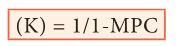• The multiplier is the reciprocal of one minus marginal propensity to consume.
• Since marginal propensity to save is 1 – MPC. (MPC+MPS =1).
• Multiplier is 1/ MPS.
• The multiplier is therefore defined as reciprocal of MPS. Multiplier is inversely related to MPS and directly with MPC.
• Numerically if MPC is 0.75, MPS is 0.25 and k is 4.
• Using formula k = 1/1- MPC 1/1-0.75 =1/0.25 =4Taking the following values, we can explain the functioning of multiplier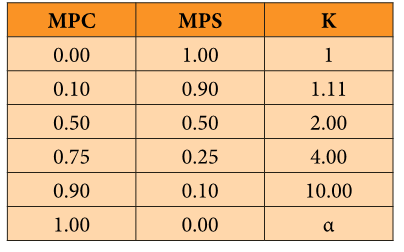C = 100 + 0.8 y; I = 100 I = 10 Y = C + I= 100 + 0.8y + 100 0.2y = 200 Y = 1000 Here, C = 100 + 0.8y = 100 + (1000) = 900; S = 100 = I After I is raised by 10, now I = 110, Y = 100 + 0.8y + 110 0.2y = 210Here C = 100=0.8(1050) = 940; S = 110 = I

Diagrammatic Explanation.

• At 45° line y = C+ S It implies the variables in axis and axis are equal.
• The MPC is assumed to be at 0.8 (C = 100 + 0.8y)
• The aggregate demand (C+I) curve intersects 45° line at point E.
• The original national income is 500. (C = 100 + 0.8y = 100 + 0.8(500) = 500) ,When I is 100,
• y = 1000, C = 900; S = 100 = I
• The new aggregate demand curve is C+I’ = 100 + 0.8y + 100 +10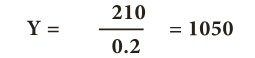• C = 940; S = 110 = I

Working of Multiplier

• Suppose the Government undertakes investment expenditure equal to Rs100 crore on some public works, by way of wages, price of materials etc.
• Thus income of labourers and suppliers of materials increases by Rs100 crore.
• Suppose the MPC is 0.8 that is 80 %. A sum of Rs80 crores is spent on consumption (A sum of Rs20 Crores is saved).
• As a result, suppliers of goods get an income of Rs80 crores. They inturn spend Rs64 crores (80% of Rs80 cr).
• In this manner consumption expenditure and increase in income act in a chain like manner.

Positive Multiplier and Negative Multiplier Effects

 Positive Multiplier Negative Multiplier When an intial increases in an injection (or a decrease in a leakage ) leads to a greater final increase in real GDP. When an intial increases in an injection (or an increase in a leakage ) leads to a greater final decrease in real GDP.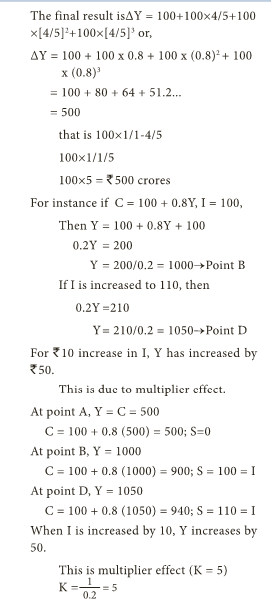Classification of Multiplier:

1. Static and dynamic multiplier

• Static multiplier is otherwise known as simultaneous multiplier, timeless multiplier, and logical multiplier.
• Under static multiplier the change in investment and the resulting change in income are simultaneous.
• There is no time lag.
• There is also no change in MPC as the economy moves from one equilibrium position to another.
• Dynamic multiplier is also known as ‘sequence multiplier’.
• In real life, income level does not increase instantly with investment.
• In fact, there is a time lag between increase in income and consumption expenditure.

Leakages of multiplier

• The multiplier assumes that those who earn income are likely to spend a proportion of their additional income on consumption.
• But in practice, people tend to spend their additional income on other items.
• Such expenses are known as leakages.

Payment towards past debts.

• If a portion of the additional income is used for repayment of old loan, the MPC is reduced and as a result the value of multiplier is cut.

Purchase of existing wealth

• If income is used in purchase of existing wealth such as land, building and shares money is circulated among people and never enters into the consumption stream.
• As a result the value of multiplier is affected.

Import of goods and services

• Income spent on imports of goods or services flows out of the country and has little chance to return to income stream in the country.
• Thus imports reduce the value of multiplier.

Non availability of consumer goods

• The multiplier theory assumes instantaneous supply of consumer goods following demand.
• But there is often a time lag.
• During this gap (D>S) inflation is likely to rise.
• This reduces the consumption expenditure and thereby multiplier value.

Full employment situation

• Under conditions of full employment, resources are almost fully employed.
• So, additional investment will lead to inflation only, rather than generation of additional real income.

Uses of multiplier

1. Multiplier highlights the importance of investment in income and employment theory.
2. The process throws light on the different stages of trade cycle.
3. It also helps in bringing the equality between S and I.
4. It helps in formulating Government policies.
5. It helps to reduce unemployment and achieve full employment.The Accelerator Principle

• The origin of accelerator principle can be traced back in the writings of Aftalion (1909), Hawtrey (1913) and Bickerdike (1914).
• However, the systematic development of the simple accelerator model was made by J.M.Clark, in 1917.
• It was further developed by Hicks, Samuelson and Harrod in relation to the business cycles.

Meaning

• A given increase in the demand for consumption goods in the economy generally leads to an accelerated demand for machineries (investment goods).
• Accelerator is the numerical value of the relation between an increase in consumption and the resulting increase in investment.
• The accelerator expresses the ratio of the net change in investment to change in consumption.

Accelerator Effects

• Increase in consumer demand
• Films get close to fill capacity
• Films invest to meet rising demand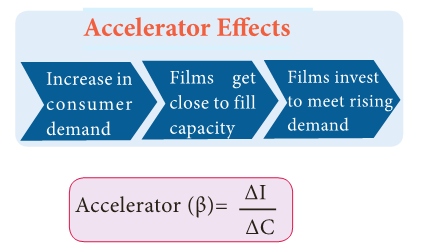Definition

• The accelerator coefficient is the ratio between induced investment and an initial change in consumption.”
• Assuming the expenditure of Rs 50crores on consumption goods, if industries lead to an investment of Rs100crores in investment goods industries, we can say that the accelerator is 2.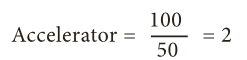Assumptions

1. Absence of excess capacity in consumer goods industries.
2. Constant capital – output ratio
3. Increase in demand is assumed to be permanent
4. Supply of funds and other inputs is quite elastic
5. Capital goods are perfectly divisible in any required size.

Operation of the Acceleration Principle

• Let us consider a simple example.
• The operation of the accelerator may be illustrated as follows.
• Let us suppose that in order to produce 1000 consumer goods, 100 machines are required.
• Also suppose that working life of a machine is 10 years.
• This means that every year 10 machines have to be replaced in order to maintain the constant flow of 1000 consumer goods.
• This might be called replacement demand.
• Suppose that demand for consumer goods rises by 10 percent (ie from 1000 to 1100). This results in increase in demand for 10 more machines.
• So that total demand for machines is 20. (10 for replacement and 10 for meeting increased demand).
• It may be noted here a 10 percent increase in demand for consumer goods causes a 100 percent increase in demand for machines (from 10 to 20).
• So we can conclude even a mild change in demand for consumer goods will lead to wide change in investment.

Diagrammatic illustration:Operation of Accelerator.

• SS is the saving curve. II is the investment curve. At point E1, the economy is in equilibrium with OY1 income.
• Saving and investment are equal at OI2. Now, investment is increased from OI2 to OI4.
• This increases income from OY1 to OY3, the equilibrium point being E3.
• If the increase in investment by I2 I4 is purely exogenous, then the increase in income by Y1 Y3 would have been due to the multiplier effect.
• But in this diagram it is assumed that exogenous investment is only by I2 I3 and induced investment is by I3 I4.
• Therefore, increase in income by Y1 Y2 is due to the multiplier effect and the increase in income by Y2 Y3 is due to the accelerator effect.

Limitations

1. The assumption of constant capital- output ratio is unrealistic.
2. Resources are available only before full employment.
3. Excess capacity in capital goods industries is assumed.
4. Accelerator will work only if the increased demand is permanent.
5. Accelerator will work only when credit is available easily.
6. If there is unused or excess capacity in the consumer goods industry, the accelerator principle would not work.

Super Multiplier: (k and ß interaction)

• In order to measure the total effect of initial investment on income, Hicks has combined the k and ß mathematically and given it the name of the Super Multiplier.
• The super multiplier is worked out by combining both induced consumption and induced investment.
• The super multiplier is greater than simple multiplier which includes only autonomous investment and no induced investment, while super multiplier includes induced investment.

Leverage Effect

• The combined effect of the multiplier and the accelerator is also called the leverage effect which may lead the economy to very high or low level of income propagation. Symbolically ,• Y = Aggregate income. C = Consumption expenditure IA= autonomous investment IP= induced private investment

MORE TO KNOW:

• The chapter consumption function and investment function can be summarised under three heads.
• The consumption function deals with relationship between national income and consumption expenditure Viz APC, MPC and APS, MPS.
• The subjective and objective factors determine consumption function.
• The investment function includes autonomous investment and the induced investment, the functional relationship between interest rate and the investment, the role of MEC and rate of interest in determining the investment.
• The multiplier is directly related to MPC and inversely related to MPS.
• The accelerator principle explains the effect of changing consumption expenditure upon volume of investment.
• The interaction of multiplier and accelerator is called super multiplier.